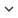ENGArticle
Article 1659
Author/s
• Constantino A. García, Paulo Félix, Jesús M. Presedo, Abraham Otero
DOI
Source
• Journal of Computational Physics, 2022

# Stochastic embeddings of dynamical phenomena through variational autoencoders

System identification in scenarios where the observed number of variables is less than the degrees of freedom in the dynamics is an important challenge. In this work we tackle this problem by using a recognition network to increase the observed space dimensionality during the reconstruction of the phase space. The phase space is forced to have approximately Markovian dynamics described by a Stochastic Differential Equation (SDE), which is also to be discovered. To enable robust learning from stochastic data we use the Bayesian paradigm and place priors on the drift and diffusion terms. To handle the complexity of learning the posteriors, a set of mean field variational approximations to the true posteriors are introduced, enabling efficient statistical inference. Finally, a decoder network is used to obtain plausible reconstructions of the experimental data. The main advantage of this approach is that the resulting model is interpretable within the paradigm of statistical physics. Our validation shows that this approach not only recovers a state space that resembles the original one, but it is also able to synthesize new time series capturing the main properties of the experimental data.
Keywords: Stochastic Differential Equation, Gaussian process state space model, Structured variational autoencoder